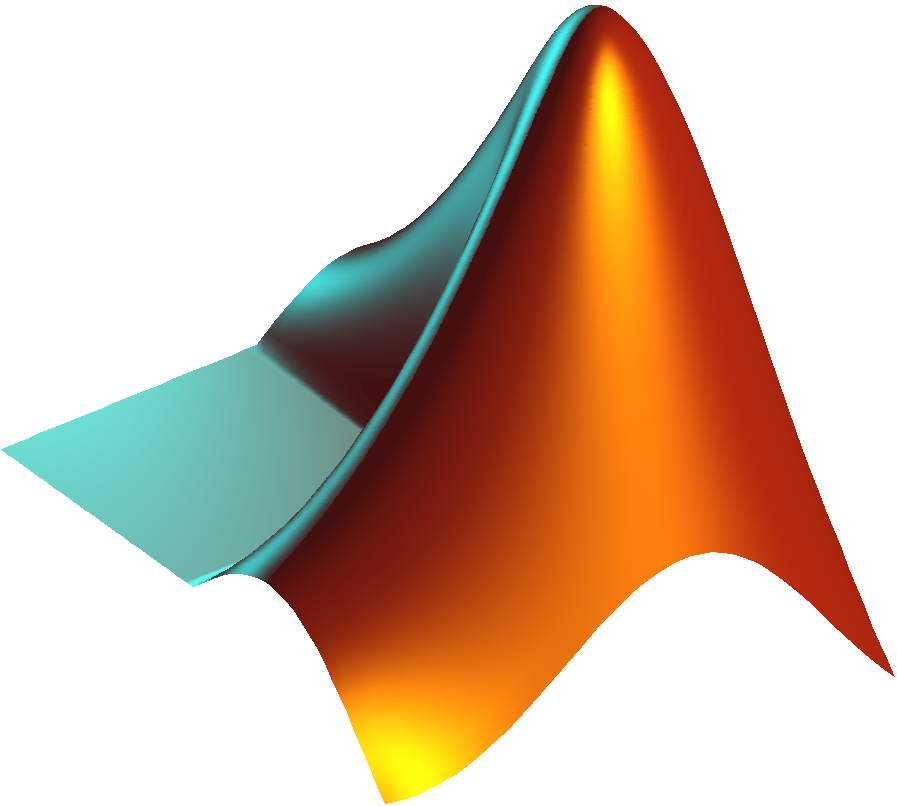##### Carbon Dioxide Density

Matlab file (32-bit system)

Determination of carbon dioxide density from the relationship proposed by Pitzer and Schreiber (1988), which provides accurate results very near the critical point.##### Carbon Dioxide Viscosity

Matlab file (32-bit system)

The viscosities of CO2 were estimated using the empirical equation developed by Altunin and Sakhabetdinov (1972). In this expression, viscosity is a function of density and temperature.##### D12 calculation

Microsoft Excel

Spreadsheet for D12 calculation, using a model, developed in this group, for real systems containing at least one polar component. It is based on the Rice and Gray approach applied to the Stockmayer potential.##### D12 calculation

Microsoft Excel

Free-volume model for tracer diffusion coefficients, D12, developed for systems in supercritical CO2 and liquid water as solvents. It embodies the concepts of free volume and activation energy.##### D12 calculation

Microsoft Excel

Universal model for accurate calculation of binary diffusivities, D12, of solutes inﬁnitely diluted in gas, liquid and supercritical solvents. It is based on a Lennard–Jones model, and contains two parameters: the molecular diameter of the solvent and a diffusion activation energy. The model is universal since it is applicable to polar, weakly polar, and non-polar solutes and/or solvents, over wide ranges of temperature and density.